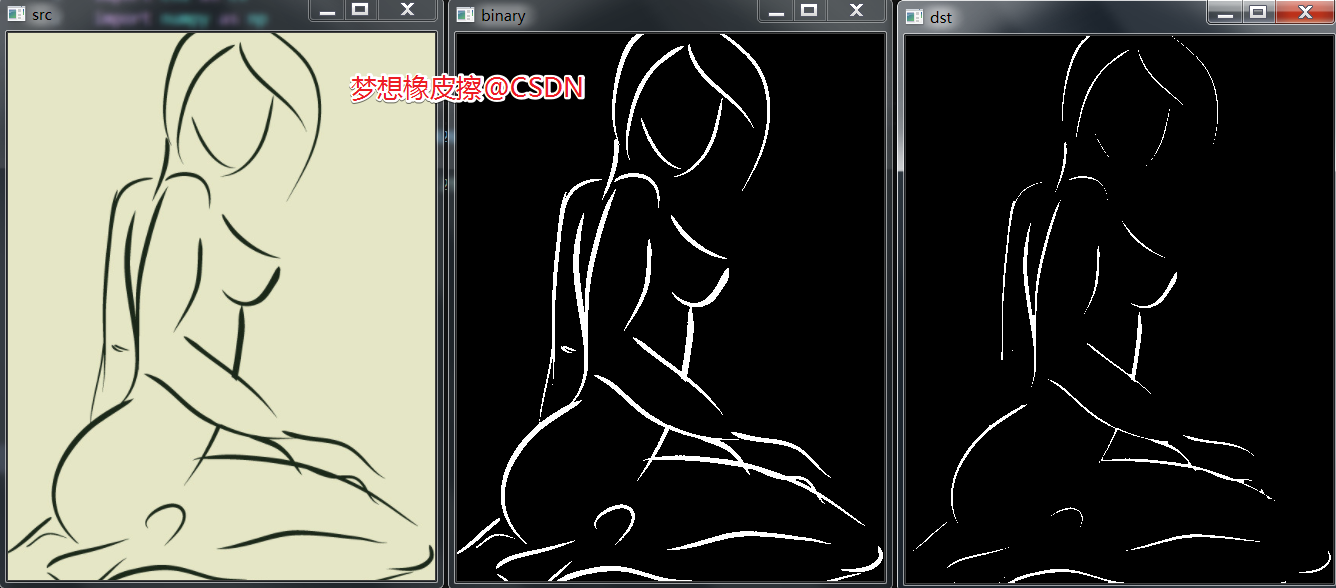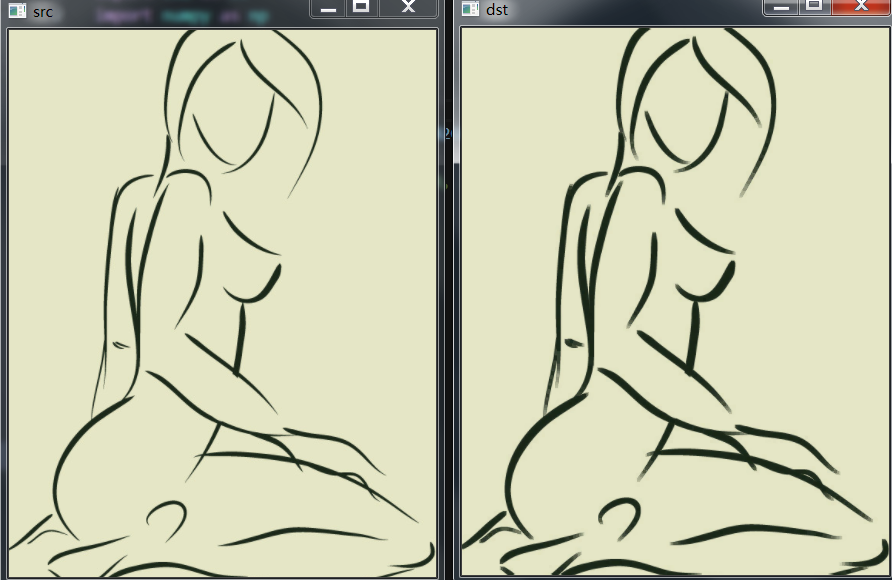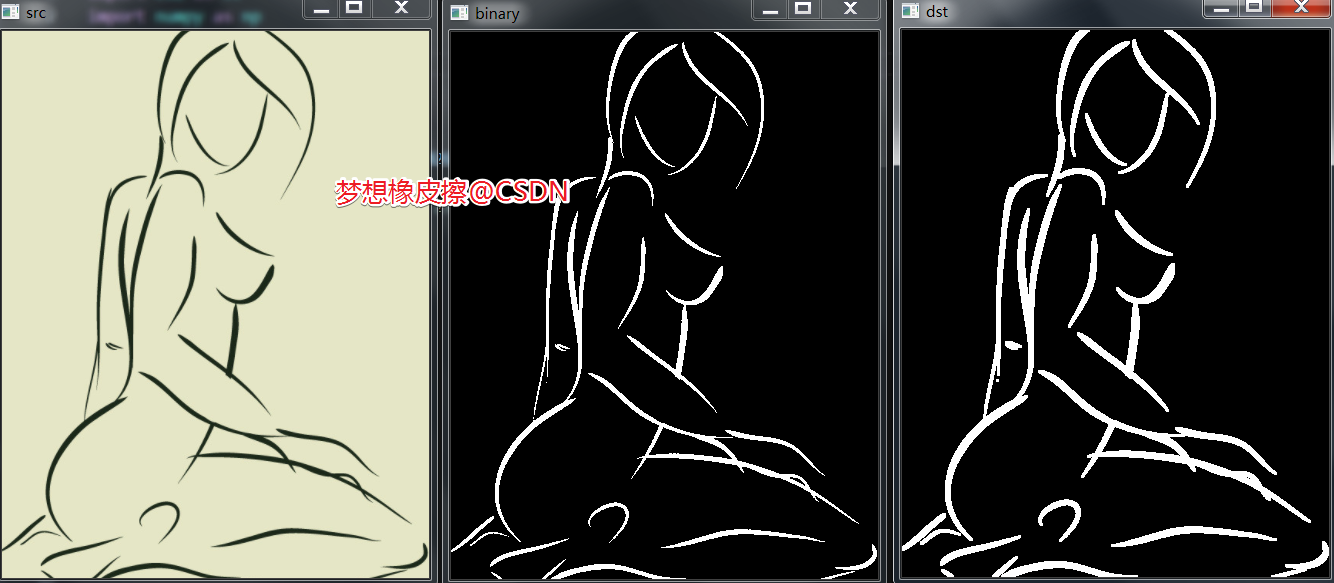# Python OpenCV 图像的膨胀与腐蚀，图像处理取经之旅第 38 篇Python OpenCV 365 天学习计划，与橡皮擦一起进入图像领域吧。本篇博客是这个系列的第 38 篇。

## 图像腐蚀（Erosion ）

### 函数原型

dst = cv2.erode(src, kernel[, dst[, anchor[, iterations[, borderType[, borderValue]]]]])

• src：待腐蚀的二值图；

• kernel：腐蚀操作的内核，默认是 3X3 矩阵，也可以使用 cv2.getStructuringElement 函数指明形状，下文具体说明；

• anchor：核矩阵锚点，不传值或传值为 (-1,-1) 则取核矩阵的中心位置作为锚点；

• iterations：腐蚀次数，默认为 1；

• borderType

kernel 为核矩阵，该结构元素可以是矩形、椭圆、十字形，都是通过 cv2.getStructuringElement 函数生成不同形状的结构元素。

• kernel = cv2.getStructuringElement(cv2.MORPH_RECT, (5, 5)) ：矩形结构；

• kernel = cv2.getStructuringElement(cv2.MORPH_ELLIPSE, (5, 5)) ：椭圆结构；

• kernel = cv2.getStructuringElement(cv2.MORPH_CROSS, (5, 5)) ：十字形结构。

import cv2 as cvimport numpy as np# 图像读取src = cv.imread("./t2.png")# 灰度图片gray = cv.cvtColor(src, cv.COLOR_BGR2GRAY)# 二值化ret, binary = cv.threshold(gray, 0, 255, cv.THRESH_BINARY_INV | cv.THRESH_OTSU)cv.imshow("binary", binary)# 卷积核kernel = np.ones((3, 3), np.uint8)# 进行图像腐蚀，默认迭代 1 次dst = cv.erode(binary, kernel)# 图像显示cv.imshow("src", src)cv.imshow("dst", dst)cv.waitKey(0)cv.destroyAllWindows()dst = cv.erode(binary, kernel,iterations=3)

## 图像膨胀（dilation ）

### 函数原型

dst = cv2.dilate(src, kernel[, dst[, anchor[, iterations[, borderType[, borderValue]]]]])

import cv2 as cvimport numpy as np# 图像读取src = cv.imread("./t2.png")# 灰度图片gray = cv.cvtColor(src, cv.COLOR_BGR2GRAY)# 二值化ret, binary = cv.threshold(gray, 0, 255, cv.THRESH_BINARY_INV | cv.THRESH_OTSU)# 卷积核kernel = np.ones((3, 3), np.uint8)# 进行图像膨胀，默认迭代 1 次dst = cv.dilate(binary, kernel)# 图像显示cv.imshow("src", src)cv.imshow("binary", binary)cv.imshow("dst", dst)cv.waitKey(0)cv.destroyAllWindows()• 对象大小增加一个像素（3x3）；

• 平滑对象边缘；

• 减少或者填充对象之间的距离。

## 橡皮擦的小节### 梦想橡皮擦

6 年产品经理+教学经验，3 年互联网项目管理经验； 互联网资深爱好者； 沉迷各种技术无法自拔，导致年龄被困在 25 岁； CSDN 爬虫 100 例作者。 个人公众号“梦想橡皮擦”。

## 评论